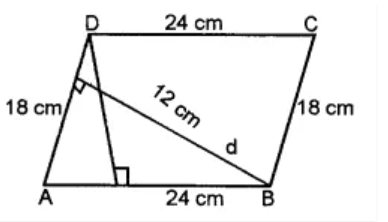# ML Aggarwal Solutions Class 8 Mathematics Solutions for Mensuration Exercise 18.1 in Chapter 18 - Mensuration

Two adjacent sides of a parallelogram are 24 cm and 18 cm. If the distance between longer sides is 12 cm, find the distance between shorter sides.Let take 24 cm as the base of a parallelogram, then its height is 12 cm.

We know that,

Area of parallelogram = base × height

= 24 × 12 = 288 cm2

Let’s consider d cm to be the distance between the shortest sides.

∴ Area of parallelogram = (18 × d) cm2

18 × d = 288

⇒ d = 288/18 = 16 cm

Therefore, the distance between the shorter sides is 16 cm.

Related Questions

Lido

Courses

Teachers

Book a Demo with us

Syllabus

Maths
CBSE
Maths
ICSE
Science
CBSE

Science
ICSE
English
CBSE
English
ICSE
Coding

Terms & Policies

Selina Question Bank

Maths
Physics
Biology

Allied Question Bank

Chemistry
Connect with us on social media!